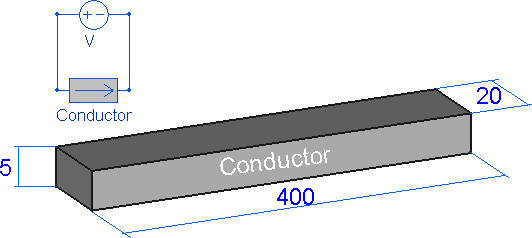QuickField

A new approach to field modelling
 Language: Global English Deutch Espanol Francais Italiano Danmark Ceske Chinese Pycckuü

Main >> Applications >> Sample problems >> Joule-Lenz law

# Joule-Lenz law

This verification example compares the current and the Joule heat generated in conductor calculated by the Ohm's law and Joule's law, and calculated in QuickField using three formulations: DC conduction, AC conduction and Transient electric.

Geometry:
All dimensions are in millimetersGiven:
V = 0.1 V - voltage applied;
f = 50 Hz - frequency in time harmonics and transient magnetics problems;
g = 1e6 S/m - conductivity of conductor material.
L = 0.4 m - conductor length.
A = 0.02*0.005 m2 - conductor cross-section area.

Calculate the current and Joule heat inside the conductor and compare with the value given by the Joule–Lenz law.

Solution:
To maintain the same value of Joule heat across all formulations the voltage value is adjusted:
In the time harmonics problem peak amplitude value of voltage is set √2·V.
In the transient electric voltage is set via formula V(t) = √2·V · sin(2·180·50·t).

According to the Joule–Lenz law* the the power of heating generated by an electrical current I:
W = R*I2, where the conductor resistance is R = (1/g) * (L/A).
The electric current value could be calculated I = V / R [A],

Results:
Conductor resistance R = (1/1e6) * (0.4/0.02*0.005) = 0.004 Ohm
Current: I = 0.1 / 0.004 = 25 A
Joule heat Q = 0.004 * 25 * 25 = 2.5 W.

DC conduction:Time harmonics (peak current value is presented, RMS value is √2 times smaller, 35.355/1.4142 = 25 A):Transient electric:
 Time Current Joule heat 0.01 s 0 A 0 W 0.0125 s 25 A 2.5 W 0.015 s 35.355 A 5 WHeat power [W] Joule-Lenz law 2.5 DC conduction 2.5 Time harmonics 2.5 Transient electric (time-average) 2.5
• Video:
• View simulation report in PDF
• Download simulation files (files may be viewed using any QuickField Edition).